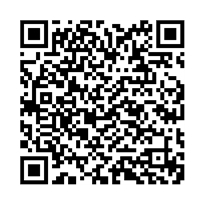#jsDisabledContent { display:none; } My Account |  Register |  HelpOpen EEWOWW Add to Book Shelf Flag as InappropriateThis book will be permanently flagged as inappropriate and made unaccessible to everyone. Are you certain this book is inappropriate?          Excessive Violence          Sexual Content          Political / Social Email this Book Email Address:

# The Distribution of Natural Numbers Divisible by 2, 3, 5, 11, 13 and 17 on the Square Root Spiral

## By Hahn, Harry, K.

Book Id: WPLBN0100301948
Format Type: PDF (eBook)
File Size: 983.24 KB.
Reproduction Date: 6/28/2007

 Title: The Distribution of Natural Numbers Divisible by 2, 3, 5, 11, 13 and 17 on the Square Root SpiralAuthor: Hahn, Harry, K. Volume: Language: English Subject: Collections: Historic Publication Date: 2007 Publisher: Self-published Member Page: Harry Hahn Citation APA MLA Chicago Hahn, H. K., & Hahn, H. K. (2007). The Distribution of Natural Numbers Divisible by 2, 3, 5, 11, 13 and 17 on the Square Root Spiral. Retrieved from http://self.gutenberg.org/

Description
The Square Root Spiral ( or “Spiral of Theodorus” or “Einstein-Spiral” ) is a very interesting geometrical structure, in which the square roots of all natural numbers have a defined (spatial ) position to each other. The Square Root Spiral develops from a right angled base triangle with the two legs ( cathets ) having the length 1, and with the long side ( hypotenuse ) having a length which is equal to the square root of 2. The Square Root Spiral is formed by further adding right angled triangles on this base triangle. In this process the longer legs of the next triangles always attach to the hypotenuses of the previous triangles. And the longer leg of the next triangle always has the same length as the hypotenuse of the previous triangle, and the shorter leg always has the length 1. In this way a spiral structure is developing in which the spiral is created by the shorter legs of the triangles, which have the constant length of 1, and where the lengths of the radial rays ( or spokes ) coming from the centre of this spiral are the square roots of the natural numbers ( sqrt 2 , sqrt 3, sqrt 4, sqrt 5 …. ). The most striking property of the Square Root Spiral is surely the fact, that the distance between two successive winds of the Square Root Spiral quickly strives for the well known geometrical constant Pi (π) !! But there are many more interesting interdependencies between the natural numbers, which can be discovered in this amazing geometrical structure. For example all numbers divisible by the same prime factor always lie on defined spiral-graphs, which run in a clear order through the Square Root Spiral. And the square numbers 4, 9, 16, 25, ... form a highly three-symmetrical sytem of three spiral-graphs, which divide the Square Root Spiral into three equal areas. It is the same with Prime Numbers. Prime Numbers also clearly accumulate on defined spiral-graphs. A mathematical analysis shows, that all these spiral graphs represent special quadratic polynomials. Even the famous Fibonacci Numbers play a part in the structure of the Square Root Spiral. Fibonacci Numbers divide the Square Root Spiral into areas and angles with constant proportions, which are linked to the “golden mean” ( or golden section ). The Square Root Spiral ( or Einstein Spiral ) shows the interdependencies between the natural numbers in a visual way. Therefore it can be considered to be a kind of visual representation of number theory ! Through pure graphical analysis of this amazing structure, the higher logic of the ( spatial ) distribution of the natural numbers ( and special sub-groups like square numbers or prime numbers ) comes to light and is very easy to grasp, because it is visible ! Please have a read through my more detailed introduction about the distribution of the natural numbers on the Square Root Spiral. This introduction gives a good insight into the interdependencies between the natural numbers, which exist in this amazing geometrical structure. Titel : The ordered distribution of the natural numbers on the Square Root Spiral

Summary
The natural numbers divisible by the Prime Factors 2, 3, 5, 11, 13 and 17 lie on defined spiral-graphs, which run through the Square Root Spiral. And a mathematical analysis shows that these spiral graphs are defined by specific quadratic polynomials. Basically all natural number which are divisible by the same prime factor lie on such spiral-graphs. And these spiral-graphs can be assigned to a certain number of “spiral graph systems” which have a defined spatial orientation to each other. This document represents a supplementation to my detailed introduction study to the Square Root Spiral, and it contains the missing diagrams and analyses for the distribution of the natural numbers divisible by 2, 3, 5, 11, 13 and 17 on the Square Root Spiral. My introduction study can be found here in this archive or in the ArXiv–archive : Title of the study : The ordered distribution of the natural numbers on the Square Root Spiral ( This study also contains diagram & analysis for numbers divisible by 7, not included in this study here ! )

Excerpt
From the image FIG 1 it is evident that the numbers divisible by 2 ( marked in yellow ) lie on defined spiral graphs which have their starting point in or near the centre of the square root spiral. These spiral graphs have either a positive or a negative rotation direction. A spiral graph which has a clockwise rotation direction shall be called negative (N) and a spiral graph which has an counterclockwise rotation direction shall be called positive (P). The green spiral-graphs show the highly three-symmetrical arrangement of the three spiral-graphs which contain the square numbers 4, 9, 16, 25, 36, 49, …. ( drawn for reference only ! )